Friday 22nd January 2021

CBSE Guess > Papers > Question Papers > Class XII > 2004 > Maths > Compartment Delhi Set-I

MATHS (Set I—Compartment Delhi)

SECTION - A

Q. 1. Using properties of determinants, Prove that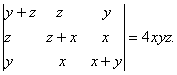Q. 2. If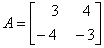, find Where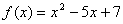Q. 3. Solve the following differential equation: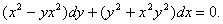Q. 4. Two dice are tossed together. Find the probability of getting a double or a total of 10.

Q. 5. Solve the differential equation: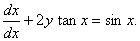Q. 6. Two cards are drawn successively at random, without replacement, from a well shuffled pack of 52 cards. Find the probability distribution number of aces.

Q. 7. Evaluate: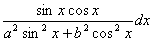Q. 8. Evaluate: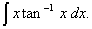Or

Evaluate: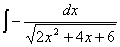Q. 9. Find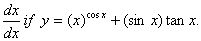Q. 10. For each x in a Boolean Algebra B, show that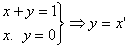Or

Prove that: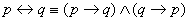Q. 11. Find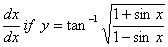Or

Differentiate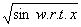from first principle.

Q. 12. Find the intervals in which the function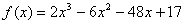is (a) increasing, (d) decreasing.

Q. 13. Evaluate: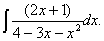Q. 14. Evaluate: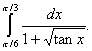Q. 15. Evaluate: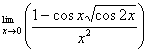Q. 16. Using matrices, solve the following system of equations: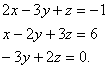Q. 17. Show that the height of cylinder at maximum volume that can be inscribed in a sphere of radius R is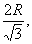Or

Find the equations of tangents to the curve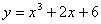which are perpendicular to the line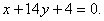Q. 18. Using integration, find the area of the region in the first quadrant enclosed by the x-axis, the line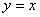and the circle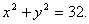SECTION - B

Q. 19. Find if the vectors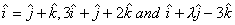are coplanar.

Q. 20. If a, b and c are the lengths of the sides opposite to the angles A, B and C respectively of a then show that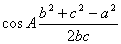Or

A particle acted on by constant forces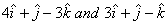displaced from the point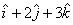to the point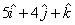. Find the total work done by the forces.

Q. 21. Show that the sphere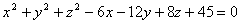touches the plane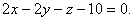Q. 22. Find the equation of a line passing through the point (2, 1, 3) and perpendicular to the lines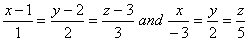,

Q. 23. Find the length of the perpendicular drawn from the point (2, 3, 7) to the plane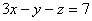. Also find the coordinates of the foot of the perpendicular.

Q. 24. A body of weight 10 n is suspended by two strings 14 cm and 48 cm long, their other ends being fastended to the end points of a rod of length 50 cm. if the rod be so held that the body hangs immediately below its mid-point, find the tensions in the string.

Q. 25. The resultant of two forces P and Q. inclined to each other at an angle a is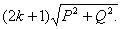when they are inclined at an angle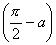the resultant is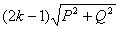show that

Q. 26. A body is projected vertically upwards with a velocity of 24.5 m/sec, where g=9.8 m/sec2 .
(i) How high will it go?
(ii) How ling will it take to return to the point of projection?
(iii) When will its velocity be 4.9 m/sec?
(iv) At particle is projected so as to graze the tops of two walls, each of height 10 m, at 15 m and 45 m respectively form the point of projection. Find the angle of projection.

Or

A particle is projected so as to graze the tops of two walls, each of height 10 m, at 15 m and 45 m respectively from the point of projection. Find the angle of projection.

SECTION - C

Q. 19. One bill of Rs. 5,000 legally due on June 13 and another for Rs. 4,000 due on August 25, are both discounted by a broker on April 1. If the difference between the two discounts is Rs. 24, find the rate of which the two discounts is Rs. 24, find the rate at which the discounted is calculated.

Q. 20. One bill of Rs.5, 000 legally due on June 13 and another for Rs. 4, 000 legally due on August 25, are both discounted by a broker on April 1. If the difference between the two discounts is Rs. 24, find the rate at which the discount is calculate.

Q. 21. Bag A contains 3 white and 2 red balls. Bag B contains 4 white and 5 red balls. One ball is drawn at random from one of the bags and is found to be red. Find the probability that it was drawn from Bag B.

Q. 22. 20% of the bulbs produced by a machine are defective. Find the probability distribution of the number of defective bulbs in a sample of 4 bulbs chosen at random.
Or
The mean and variance of a binomial distribution are 12 and 3 respectively bulbs in a sample of 4 bulbs chose at random.

Q. 23. Two partners A and B invested Rs. 1,25,000 and Rs.85,000 respectively in a business. They decided to share 60% of the total profit equally and the remaining in the ratio of their investment. If A received Rs. 3,200 more than B, find the total profit earned by them.

Q. 24. A machine costs a company Rs. 97,000 and its effective life is estimated to be 12 years. If its scrap value is Rs. 2,000 only, what amount should be retained out of the profits at the end of each year to meet the expenses of the machine in time, when the rate of compound interest is 5% per annum?

Q. 25. If the total cost function of a product is given by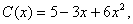where x is the output,
Prove that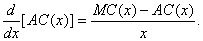Q. 26. Two tailors A and B earn Rs. 15 and Rs. 20 per day respectively. A can stitch 6 shirts and 4 pants per day while B can stitch 10 shirts and 4 pants per day. How many days shall each work, if it is desired to produce at least 60 shirts and 32 pants at a minimum labour cost? Solve the problem graphically.

Or

Anil wants to invest at most Rs. 12, 000 in bonds A and B. According to the rules, he has to invest at least Rs. 2, 000 in bond A and at least Rs. 4, 000 in Bond B. If the rate of interest on Bond A is 8% per annum and on Bond B is 10% per annum. How should he invest his money for maximum interest? Solve the problem graphically.

 Maths 2004 Question Papers Class XII Delhi Outside Delhi Compartment Delhi Compartment Outside DelhiSet ISet ISet ISet ISet IISet IISet IISet IISet IIISet III

CBSE 2004 Question Papers Class XII